# Optimal regular differential operators with variable coefficients and applications

## Abstract

In this paper, maximal regularity properties for linear and nonlinear high-order elliptic differential-operator equations with VMO coefficients are studied. For the linear case, the uniform coercivity property of parameter-dependent boundary value problems is obtained in ${L}^{p}$ spaces. Then, the existence and uniqueness of a strong solution of the boundary value problem for a high-order nonlinear equation are established. In application, the maximal regularity properties of the anisotropic elliptic equation and the system of equations with VMO coefficients are derived.

AMS Subject Classification:58I10, 58I20, 35Bxx, 35Dxx, 47Hxx, 47Dxx.

## 1 Introduction

The goal of the present paper is to study the nonlocal boundary value problems (BVPs) for parameter-dependent linear differential-operator equations (DOEs) with discontinuous top-order coefficients

$sa\left(x\right){u}^{\left(2m\right)}\left(x\right)+A\left(x\right)u\left(x\right)+\sum _{k=0}^{2m-1}{s}^{\frac{k}{2m}}{A}_{k}\left(x\right){u}^{\left(k\right)}\left(x\right)+\lambda u\left(x\right)=f\left(x\right),$
(1)

and the nonlinear equation

$a\left(x\right){u}^{\left(2m\right)}\left(x\right)+B\left(x,u,{u}^{\left(1\right)},\dots ,{u}^{\left(2m-1\right)}\right)u\left(x\right)=F\left(x,u,{u}^{\left(1\right)},\dots ,{u}^{\left(2m-1\right)}\right),$

where a is a complex-valued function, s is a positive and λ is a complex parameter; $A=A\left(x\right)$, ${A}_{k}={A}_{k}\left(x\right)$ are linear and B is a nonlinear operator in a Banach space E. Here the principal coefficients a and A may be discontinuous. More precisely, we assume that a and $A\left(\cdot \right){A}^{-1}\left({x}_{0}\right)$ belong to the operator-valued Sarason class VMO (vanishing mean oscillation). Sarason class VMO was at first defined in . In the recent years, there has been considerable interest to elliptic and parabolic equations with VMO coefficients. This is mainly due to the fact that VMO spaces contain as a subspace $C\left(\overline{\mathrm{\Omega }}\right)$ that ensures the extension of ${L}_{p}$-theory of operators with continuous coefficients to discontinuous coefficients (see, e.g., ). On the other hand, the Sobolev spaces ${W}^{1,n}\left(\mathrm{\Omega }\right)$ and ${W}^{\sigma ,\frac{\sigma }{n}}\left(\mathrm{\Omega }\right)$, $0<\sigma <1$, are also contained in VMO. Global regularity of the Dirichlet problem for elliptic equations with VMO coefficients has been studied in . We refer to the survey , where excellent presentation and relations with similar results can be found concerning the regularizing properties of these operators in the framework of Sobolev spaces.

It is known that many classes of PDEs (partial differential equations), pseudo DEs (differential equations) and integro DEs can be expressed in the form of DOEs. Many researchers (see, e.g., ) investigated similar spaces of functions and classes of PDEs under a single DOE. Moreover, the maximal regularity properties of DOEs with continuous coefficients were studied, e.g., in [12, 14, 18, 19].

Here the equation with top-order VMO-operator coefficients is considered in abstract spaces. We will prove the uniform separability of the problem (1), i.e., we show that for each $f\in {L}^{p}\left(0,1;E\right)$, there exists a unique strong solution u of the problem (1) and a positive constant C depending only p, E, m and A such that

$\sum _{i=0}^{2m}{s}^{\frac{i}{2m}}|\lambda {|}^{1-\frac{i}{2m}}{\parallel {u}^{\left(i\right)}\parallel }_{{L}^{p}\left(0,1;E\right)}+{\parallel Au\parallel }_{{L}^{p}\left(0,1;E\right)}\le C{\parallel f\parallel }_{{L}^{p}\left(0,1;E\right)}.$

Note that the principal part of a corresponding differential operator is non self-adjoint. Nevertheless, the sharp uniform coercive estimate for the resolvent and Fredholmness are established. Then, the existence and uniqueness of the above nonlinear problem are derived. In application, we study maximal regularity properties of anisotropic elliptic equations in mixed ${L}^{p}$ spaces and systems (finite or infinite) of differential equations with VMO coefficients in the scalar ${L}^{p}$ space.

Since (1) involves unbounded operators, it is not easy to get representation for the Green function and the estimate of solutions. Therefore we use the modern harmonic analysis elements, e.g., the Hilbert operators and the commutator estimates in E-valued ${L}^{p}$ spaces, embedding theorems of Sobolev-Lions spaces and semigroup estimates to overcome these difficulties. Moreover, we also use our previous results on equations with continuous leading coefficients and the perturbation theory of linear operators to obtain main assertions.

## 2 Notations and background

Throughout the paper, we set E a Banach space and $\mathrm{\Omega }\subset {R}^{n}$. ${L}^{p}\left(\mathrm{\Omega };E\right)$ denotes the space of all strongly measurable E-valued functions that are defined on Ω with the norm

${\parallel f\parallel }_{p}={\parallel f\parallel }_{{L}^{p}\left(\mathrm{\Omega };E\right)}={\left({\int }_{\mathrm{\Omega }}{\parallel f\left(x\right)\parallel }_{E}^{p}\phantom{\rule{0.2em}{0ex}}dx\right)}^{\frac{1}{p}},\phantom{\rule{1em}{0ex}}1\le p<\mathrm{\infty }.$

$\mathit{BMO}\left(E\right)$ (bounded mean oscillation, see [25, 26]) is the space of all E-valued local integrable functions with the norm

$\underset{B}{sup}{\int }_{B}{\parallel f\left(x\right)-{f}_{B}\parallel }_{E}\phantom{\rule{0.2em}{0ex}}dx={\parallel f\parallel }_{\ast ,E}<\mathrm{\infty },$

where B ranges in the class of the balls in ${R}^{n}$, $|B|$ is the Lebesgue measure of B and ${f}_{B}$ is the average $\frac{1}{|B|}{\int }_{B}f\left(x\right)\phantom{\rule{0.2em}{0ex}}dx$.

For $f\in \mathit{BMO}\left(E\right)$ and $r>0$, we set

$\underset{\rho \le r}{sup}{\int }_{B}{\parallel f\left(x\right)-{f}_{B}\parallel }_{E}\phantom{\rule{0.2em}{0ex}}dx=\eta \left(r\right),$

where B ranges in the class of balls with radius ρ.

We will say that a function $f\in \mathit{BMO}\left(E\right)$ is in $\mathit{VMO}\left(E\right)$ if ${lim}_{r\to +0}\eta \left(r\right)=0$. We will call $\eta \left(r\right)$ the VMO modulus of f.

Note that if $E=C$, where C is the set of complex numbers, then $\mathit{BMO}\left(E\right)$ and $\mathit{VMO}\left(E\right)$ coincide with John-Nirenberg class BMO and Sarason class VMO, respectively.

The Banach space E is called a UMD-space if the Hilbert operator

$\left(Hf\right)\left(x\right)=\underset{\epsilon \to 0}{lim}{\int }_{|x-y|>\epsilon }\frac{f\left(y\right)}{x-y}\phantom{\rule{0.2em}{0ex}}dy$

is bounded in ${L}_{p}\left(R,E\right)$, $p\in \left(1,\mathrm{\infty }\right)$ (see, e.g., ). UMD spaces include, e.g., ${L}_{p}$, ${l}_{p}$ spaces and Lorentz spaces ${L}_{pq}$, $p,q\in \left(1,\mathrm{\infty }\right)$.

Let

${S}_{\phi }=\left\{\lambda \in C,|arg\lambda |\le \phi \right\}\cup \left\{0\right\},\phantom{\rule{1em}{0ex}}0\le \phi <\pi .$

A linear operator A is said to be φ-positive (or positive) in a Banach space E with bound $M>0$ if $D\left(A\right)$ is dense on E and

${\parallel {\left(A+\lambda I\right)}^{-1}\parallel }_{L\left(E\right)}\le M{\left(1+|\lambda |\right)}^{-1}$

for $\lambda \in {S}_{\phi }$, $\phi \in \left(0,\pi \right]$, I is an identity operator in E and $L\left(E\right)$ is the space of bounded linear operators in E. Sometimes $A+\lambda I$ will be written as $A+\lambda$ and denoted by ${A}_{\lambda }$. It is known [, §1.15.1] that there exist fractional powers ${A}^{\theta }$ of the positive operator A. Let $E\left({A}^{\theta }\right)$ denote the space $D\left({A}^{\theta }\right)$ with the graphical norm

${\parallel u\parallel }_{E\left({A}^{\theta }\right)}={\left({\parallel u\parallel }^{p}+{\parallel {A}^{\theta }u\parallel }^{p}\right)}^{\frac{1}{p}},\phantom{\rule{1em}{0ex}}1\le p<\mathrm{\infty },-\mathrm{\infty }<\theta <\mathrm{\infty }.$

Let ${E}_{1}$ and ${E}_{2}$ be two Banach spaces. A set $W\subset L\left({E}_{1},{E}_{2}\right)$ is called R-bounded (see [14, 23]) if there is a positive constant C such that for all ${T}_{1},{T}_{2},\dots ,{T}_{m}\in W$ and ${u}_{1,}{u}_{2},\dots ,{u}_{m}\in {E}_{1}$, $m\in N$,

${\int }_{0}^{1}{\parallel \sum _{j=1}^{m}{r}_{j}\left(y\right){T}_{j}{u}_{j}\parallel }_{{E}_{2}}\phantom{\rule{0.2em}{0ex}}dy\le C{\int }_{0}^{1}{\parallel \sum _{j=1}^{m}{r}_{j}\left(y\right){u}_{j}\parallel }_{{E}_{1}}\phantom{\rule{0.2em}{0ex}}dy,$

where $\left\{{r}_{j}\right\}$ is a sequence of independent symmetric $\left\{-1,1\right\}$-valued random variables on $\left[0,1\right]$.

Let $S\left({R}^{n};E\right)$ denote the Schwartz class, i.e., the space of all E-valued rapidly decreasing smooth functions on ${R}^{n}$. Let F denote the Fourier transformation. A function $\mathrm{\Psi }\in {L}^{\mathrm{\infty }}\left({R}^{n};B\left({E}_{1},{E}_{2}\right)\right)$ is called a Fourier multiplier from ${L}_{p}\left({R}^{n};{E}_{1}\right)$ to ${L}_{p}\left({R}^{n};{E}_{2}\right)$ if the map $u\to {\mathrm{\Lambda }}_{\mathrm{\Psi }}u={F}^{-1}\mathrm{\Psi }\left(\xi \right)Fu$, $u\in S\left({R}^{n};{E}_{1}\right)$ is well defined and extends to a bounded linear operator

${\mathrm{\Lambda }}_{\mathrm{\Psi }}:{L}_{p}\left({R}^{n};{E}_{1}\right)\to {L}_{p}\left({R}^{n};{E}_{2}\right).$

The set of all multipliers from ${L}_{p}\left({R}^{n};{E}_{1}\right)$ to ${L}_{p}\left({R}^{n};{E}_{2}\right)$ will be denoted by ${M}_{p}^{p}\left({E}_{1},{E}_{2}\right)$. For ${E}_{1}={E}_{2}=E$, it will be denoted by ${M}_{p}^{p}\left(E\right)$.

Let

${U}_{n}=\left\{\beta =\left({\beta }_{1},{\beta }_{2},\dots ,{\beta }_{n}\right)\in {N}^{n}:{\beta }_{k}\in \left\{0,1\right\}\right\}.$

Definition 1 A Banach space E is said to be a space satisfying a multiplier condition if for any $\mathrm{\Psi }\in {C}^{\left(n\right)}\left({R}^{n};L\left(E\right)\right)$, the R-boundedness of the set $\left\{{\xi }^{\beta }{D}_{\xi }^{\beta }\mathrm{\Psi }\left(\xi \right):\xi \in {R}^{n}\mathrm{\setminus }0,\beta \in {U}_{n}\right\}$ implies that Ψ is a Fourier multiplier in ${L}_{p}\left({R}^{n};E\right)$, i.e., $\mathrm{\Psi }\in {M}_{p}^{p}\left(E\right)$ for any $p\in \left(1,\mathrm{\infty }\right)$.

Definition 2 The φ-positive operator A is said to be an R-positive in a Banach space E if there exists $\phi \in \left[0,\pi \right)$ such that the set

${L}_{A}=\left\{A{\left(A+\lambda \right)}^{-1}:\lambda \in {S}_{\phi }\right\}$

is R-bounded.

A linear operator $A\left(x\right)$ is said to be positive in E uniformly in x if $D\left(A\left(x\right)\right)$ is independent of x, $D\left(A\left(x\right)\right)$ is dense in E and

$\parallel {\left(A\left(x\right)+\lambda \right)}^{-1}\parallel \le M{\left(1+|\lambda |\right)}^{-1}$

for all $\lambda \in S\left(\phi \right)$, $\phi \in \left[0,\pi \right)$.

Let ${\sigma }_{\mathrm{\infty }}\left({E}_{1},{E}_{2}\right)$ denote the space of all compact operators from ${E}_{1}$ to ${E}_{2}$. For ${E}_{1}={E}_{2}=E$, it is denoted by ${\sigma }_{\mathrm{\infty }}\left(E\right)$. Assume ${E}_{0}$ and E are two Banach spaces and ${E}_{0}$ is continuously and densely embedded into E. Let m be a natural number. ${W}^{m,p}\left(\mathrm{\Omega };{E}_{0},E\right)$ (the so-called Sobolev-Lions type space) denotes a space of all functions $u\in {L}^{p}\left(\mathrm{\Omega };{E}_{0}\right)$ possessing the generalized derivatives ${D}_{k}^{m}u=\frac{{\partial }^{m}u}{\partial {x}_{k}^{m}}$ such that ${D}_{k}^{m}u\in {L}^{p}\left(\mathrm{\Omega };E\right)$ is endowed with the norm

${\parallel u\parallel }_{{W}^{m,p}\left(\mathrm{\Omega };{E}_{0},E\right)}={\parallel u\parallel }_{{L}^{p}\left(\mathrm{\Omega };{E}_{0}\right)}+\sum _{k=1}^{n}{\parallel {D}_{k}^{m}u\parallel }_{{L}^{p}\left(\mathrm{\Omega };E\right)}<\mathrm{\infty }.$

For ${E}_{0}=E$ the space ${W}^{m,p}\left(\mathrm{\Omega };{E}_{0},E\right)$ will be denoted by ${W}^{m,p}\left(\mathrm{\Omega };E\right)$. It is clear to see that

${W}^{m,p}\left(\mathrm{\Omega };{E}_{0},E\right)={W}^{m,p}\left(\mathrm{\Omega };E\right)\cap {L}^{p}\left(\mathrm{\Omega };{E}_{0}\right).$

Let s be a positive parameter. We define in ${W}^{m,p}\left(\mathrm{\Omega };{E}_{0},E\right)$ the following parameterized norm:

${\parallel u\parallel }_{{W}_{s}^{m,p}\left(\mathrm{\Omega };{E}_{0},E\right)}={\parallel u\parallel }_{{L}^{p}\left(\mathrm{\Omega };{E}_{0}\right)}+\sum _{k=1}^{n}{\parallel s{D}_{k}^{m}u\parallel }_{{L}^{p}\left(\mathrm{\Omega };E\right)}.$

Function $u\in {W}^{2,p}\left(0,1;E\left(A\right),E,{L}_{k}\right)=\left\{u\in {W}^{2,p}\left(0,1;E\left(A\right),E\right),{L}_{k}u=0\right\}$ satisfying equation (1) a.e. on $\left(0,1\right)$ is said to be a solution of the problem (1) on $\left(0,1\right)$.

From  we have the following theorem.

Theorem A1 Suppose the following conditions are satisfied:

1. (1)

E is a Banach space satisfying the multiplier condition with respect to $p\in \left(1,\mathrm{\infty }\right)$ and A is an R-positive operator in E;

2. (2)

$\alpha =\left({\alpha }_{1},{\alpha }_{2},\dots ,{\alpha }_{n}\right)$ are n-tuples of nonnegative integer numbers such that

$\varkappa =\frac{|\alpha |}{m}\le 1\phantom{\rule{1em}{0ex}}\mathit{\text{and}}\phantom{\rule{1em}{0ex}}0<\mu \le 1-\varkappa ;$
3. (3)

$\mathrm{\Omega }\in {R}^{n}$ is a region such that there exists a bounded linear extension operator from ${W}^{m,p}\left(\mathrm{\Omega };E\left(A\right),E\right)$ to ${W}^{m,p}\left({R}^{n};E\left(A\right),E\right)$.

Then the embedding

${D}^{\alpha }{W}^{m,p}\left(\mathrm{\Omega };E\left(A\right),E\right)\subset {L}^{p}\left(\mathrm{\Omega };E\left({A}^{1-\varkappa -\mu }\right)\right)$

is continuous and there exists a positive constant ${C}_{\mu }$ such that

${s}^{\frac{|\alpha |}{m}}{\parallel {D}^{\alpha }u\parallel }_{{L}^{p}\left(\mathrm{\Omega };E\left({A}^{1-\varkappa -\mu }\right)\right)}\le {C}_{\mu }\left[{h}^{\mu }{\parallel u\parallel }_{{W}_{s}^{m,p}\left(\mathrm{\Omega };E\left(A\right),E\right)}+{h}^{-\left(1-\mu \right)}{\parallel u\parallel }_{{L}^{p}\left(\mathrm{\Omega };E\right)}\right]$

for all $u\in {W}^{m,p}\left(\mathrm{\Omega };E\left(A\right),E\right)$ and $0.

Theorem A2 Suppose all conditions of Theorem A1 are satisfied. Assume Ω is a bounded region in ${R}^{n}$ and ${A}^{-1}\in {\sigma }_{\mathrm{\infty }}\left(E\right)$. Then, for $0<\mu \le 1-\varkappa$, the embedding

${D}^{\alpha }{W}^{m,p}\left(\mathrm{\Omega };E\left(A\right),E\right)\subset {L}^{p}\left(\mathrm{\Omega };E\left({A}^{1-\varkappa -\mu }\right)\right)$

is compact.

In a similar way as in [, Theorem 2.1], we have the following result.

Lemma A1 Let E be a Banach space and $f\in \mathit{VMO}\left(E\right)$. The following conditions are equivalent:

1. (1)

$f\in \mathit{VMO}\left(E\right)$;

2. (2)

f is in the BMO closure of the set of uniformly continuous functions which belong to VMO;

3. (3)

${lim}_{y\to 0}{\parallel f\left(x-y\right)-f\left(x\right)\parallel }_{\ast ,E}=0$.

For $f\in {L}^{p}\left(\mathrm{\Omega };E\right)$, $p\in \left(1,\mathrm{\infty }\right)$, $a\in {L}^{\mathrm{\infty }}\left({R}^{n}\right)$, consider the commutator operator

$H\left[a,f\right]\left(x\right)=a\left(x\right)Hf\left(x\right)-H\left(af\right)\left(x\right)=\underset{\epsilon \to 0}{lim}{\int }_{|x-y|>\epsilon ,}\frac{\left[a\left(x\right)-a\left(y\right)\right]}{x-y}f\left(y\right)\phantom{\rule{0.2em}{0ex}}dy.$

Proof Indeed, we observe that if $f\in \mathit{VMO}\left(E\right)$ with VMO modulus η, there exists a constant C such that ${\parallel f\left(x-y\right)-f\left(x\right)\parallel }_{\ast ,E}\le C\eta \left(r\right)$ for $\parallel y\parallel \le r$ so that the E-valued usual mollifiers converge to f in the BMO norm. More precisely, given $f\in \mathit{VMO}\left(E\right)$ with VMO modulus $\eta \left(r\right)$, we can find a sequence of E-valued ${C}^{\mathrm{\infty }}$ functions $\left\{{f}_{h}\right\}$ converging to f in E-valued BMO spaces as $h\to 0$ with VMO moduli ${\eta }_{h}$ such that ${\eta }_{h}\le \eta \left(r\right)$. In a similar way, other cases are derived. □

From [, Theorem 1] and [, Corollary 2.7], we have the following.

Theorem A3 Let E be a UMD space and $a\in \mathit{VMO}\cap {L}^{\mathrm{\infty }}\left({R}^{n}\right)$. Then $H\left[a,f\right]$ is a bounded operator in ${L}^{p}\left(R;E\right)$, $p\in \left(1,\mathrm{\infty }\right)$.

From Theorem A3 and the property (2) of Lemma A1, we obtain, respectively:

Theorem A4 Assume all conditions of Theorem A3 are satisfied. Also, let $a\in \mathit{VMO}\cap {L}^{\mathrm{\infty }}\left({R}^{n}\right)$ and let η be the VMO modulus of a. Then, for any $\epsilon >0$, there exists a positive number $\delta =\delta \left(\epsilon ,\eta \right)$ such that

${\parallel H\left[a,f\right]\parallel }_{{L}^{p}\left(0,r;E\right)}\le M\epsilon {\parallel f\parallel }_{{L}^{p}\left(0,r;E\right)},\phantom{\rule{1em}{0ex}}r\in \left(0,\delta \right).$

Theorem A5 Let E be a UMD space, $p\in \left(1,\mathrm{\infty }\right)$ and $A\left(\cdot \right)$ uniformly R-positive in E. Moreover, let $A\left(\cdot \right){A}^{-1}\left({x}_{0}\right)\in {L}_{\mathrm{\infty }}\left(R;L\left(E\right)\right)\cap \mathit{BMO}\left(L\left(E\right)\right)$, ${x}_{0}\in R$. Then the following commutator operator is bounded in ${L}^{p}\left(R;E\right)$:

$\begin{array}{rcl}H\left[A,f\right]\left(x\right)& =& A\left(x\right){A}^{-1}\left({x}_{0}\right)Hf\left(x\right)-H\left(A\left(x\right){A}^{-1}\left({x}_{0}\right)f\right)\left(x\right)\\ =& \underset{\epsilon \to 0}{lim}{\int }_{|x-y|>\epsilon ,}\frac{\left[A\left(x\right){A}^{-1}\left({x}_{0}\right)-A\left(y\right){A}^{-1}\left({x}_{0}\right)\right]}{x-y}f\left(y\right)\phantom{\rule{0.2em}{0ex}}dy.\end{array}$

Note that singular integral operators in E-valued ${L}^{p}$ spaces were studied, e.g., in .

Theorem A6 Assume all conditions of Theorem A5 are satisfied and η is a VMO modulus of $A\left(\cdot \right){A}^{-1}\left({x}_{0}\right)$.

Then, for any $\epsilon >0$, there exists a positive number $\delta =\delta \left(\epsilon ,\eta \right)$ such that

${\parallel H\left[A,f\right]\parallel }_{{L}^{p}\left({\mathrm{\Omega }}_{r};E\right)}\le M\epsilon {\parallel f\parallel }_{{L}^{p}\left({\mathrm{\Omega }}_{r};E\right)},\phantom{\rule{1em}{0ex}}r\in \left(0,\delta \right).$

Consider the nonlocal BVP for parameter-dependent DOE with constant coefficients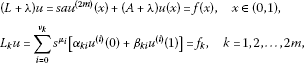(2)

where ${\nu }_{k}\in \left\{0,1,\dots ,2m-1\right\}$, a, ${\alpha }_{ki}$, ${\beta }_{ki}$ are complex numbers, ${\mu }_{i}=\frac{i}{2m}+\frac{1}{2mp}$, ${\theta }_{k}=\frac{{\nu }_{k}}{2m}+\frac{1}{2mp}$, s is a positive and λ is a complex parameter; ${A}_{\lambda }=A+\lambda$ and A is a linear operator in E. Let ${\omega }_{1},{\omega }_{2},\dots ,{\omega }_{2m}$ be roots of the equation $a{\omega }^{2m}+1=0$, $\left[{\upsilon }_{ij}\right]$ be a 2m-dimensional matrix and $\eta =|\left[{\upsilon }_{ij}\right]|$ be a determinant of the matrix $\left[{\upsilon }_{ij}\right]$, where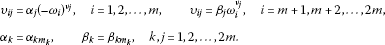It is known that (see, e.g., [, §1.15]) if the operator A is φ-positive in E, then operators ${\omega }_{k}{s}^{-\frac{1}{2m}}{A}_{\lambda }^{\frac{1}{2m}}$, $k=1,2,\dots ,2m$ generate the following analytic semigroups: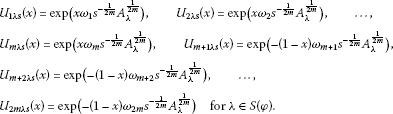Let

${E}_{k}={\left(E\left(A\right),E\right)}_{{\theta }_{k},p}.$

From [, Theorem 1] and [, Theorem 3.2], we obtain the following.

Theorem A7 Assume the following conditions are satisfied:

1. (1)

E is a Banach space satisfying the multiplier condition with respect to $p\in \left(1,\mathrm{\infty }\right)$;

2. (2)

A is an R-positive operator in E for $0\le \phi <\pi$ and $\eta \ne 0$;

3. (3)

$|arg{\omega }_{j}-\pi |\le \frac{\pi }{2}-\phi$, $j=1,2,\dots ,m$, $|arg{\omega }_{j}|\le \frac{\pi }{2}-\phi$, $j=m+1,\dots ,2m$ and $\frac{\lambda }{{\omega }_{j}}\in S\left(\phi \right)$.

Then

1. (1)

for $f\in {L}_{p}\left(0,1;E\right)$, ${f}_{k}\in {E}_{k}$, $\lambda \in S\left(\phi \right)$ and for sufficiently large $|\lambda |$, the problem (2) has a unique solution $u\in {W}^{2m,p}\left(0,1;E\left(A\right),E\right)$. Moreover, the following coercive uniform estimate holds:

$\sum _{i=0}^{2m}{s}^{\frac{i}{2m}}|\lambda {|}^{1-\frac{i}{2m}}{\parallel {u}^{\left(i\right)}\parallel }_{{L}^{p}\left(0,1;E\right)}+{\parallel Au\parallel }_{{L}^{p}\left(0,1;E\right)}\le C\left[{\parallel f\parallel }_{{L}^{p}\left(0,1;E\right)}+\sum _{k=1}^{2m}{\parallel {f}_{k}\parallel }_{{E}_{k}}\right].$
2. (2)

For ${f}_{k}=0$, the solution is represented as

$\begin{array}{rcl}u\left(x\right)& =& {\int }_{0}^{1}{G}_{\lambda s}\left(x,y\right)f\left(y\right)\phantom{\rule{0.2em}{0ex}}dy,{G}_{\lambda s}\left(x,y\right)\\ =& \sum _{k=1}^{2m}\sum _{j=1}^{2m}\sum _{i=0}^{{\nu }_{k}}\left[{B}_{kij}\left(\lambda \right){\left({s}^{-1}{A}_{\lambda }\right)}^{-\frac{1}{2m}\left(2m+{\nu }_{k}-i-1\right)}{U}_{j\lambda s}\left(x\right){U}_{k\lambda s}\left(1-y\right)\right]+{U}_{0\lambda s}\left(x-y\right),\end{array}$
(3)

where ${B}_{kij}\left(\lambda \right)$ are uniformly bounded operators in E and

${U}_{0\lambda s}\left(x-y\right)=\left\{\begin{array}{c}{a}^{-1}\left\{{s}^{1-\frac{1}{2m}}{A}_{\lambda }^{-\left(1-\frac{1}{2m}\right)}{\sum }_{i=1}^{m}{\left(-1\right)}^{2m+i}{P}_{i}^{-1}{U}_{i\lambda s}\left(x-y\right),x\ge y\right\},\hfill \\ -{a}^{-1}\left\{{s}^{1-\frac{1}{2m}}{A}_{\lambda }^{-\left(1-\frac{1}{2m}\right)}{\sum }_{i=m+1}^{2m}{\left(-1\right)}^{2m+i}{P}_{i}^{-1}{U}_{i\lambda s}\left(x-y\right),x\le y\right\},\hfill \end{array}$

where

${P}_{i}=\left({\omega }_{i}-{\omega }_{1}\right)\cdots \left({\omega }_{i}-{\omega }_{i-1}\right)\left({\omega }_{i+1}-{\omega }_{i}\right)\cdots \left({\omega }_{2m}-{\omega }_{i}\right),\phantom{\rule{1em}{0ex}}i=1,2,\dots ,2m.$

Consider the BVP for DOE with variable coefficients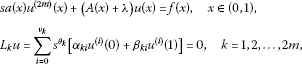(4)

where $a=a\left(x\right)$ is a complex-valued function, ${m}_{k}\in \left\{0,1,\dots ,2m-1\right\}$, ${\alpha }_{ki}$, ${\beta }_{ki}$ are complex numbers, s is a positive and λ is a complex parameter, ${\theta }_{k}=\frac{{\nu }_{k}}{2m}+\frac{1}{2mp}$ and $A\left(x\right)$ is a linear operator in E.

Let ${\omega }_{1}={\omega }_{1}\left(x\right)$, ${\omega }_{2}={\omega }_{2}\left(x\right),\dots ,{\omega }_{2m}={\omega }_{2m}\left(x\right)$ be roots of the equation $a\left(x\right){\omega }^{2m}+1=0$, $\left[{\upsilon }_{ij}\right]$ be a 2m-dimensional matrix and $\eta \left(x\right)=|\left[{\upsilon }_{ij}\right]|$ be a determinant of the function matrix $\left[{\upsilon }_{ij}\right]$, where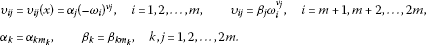In the next theorem, we consider the case when principal coefficients are continuous. The well-posedness of this problem occurs in studying of equations with VMO coefficients. From [, Theorem 3] and [, Theorem 3.2], we get the following.

Theorem A8 Suppose the following conditions are satisfied:

1. (1)

E is a Banach space satisfying the multiplier condition with respect to $p\in \left(1,\mathrm{\infty }\right)$;

2. (2)

$|arg{\omega }_{j}-\pi |\le \frac{\pi }{2}-\phi$, $j=1,2,\dots ,m$, $|arg{\omega }_{j}|\le \frac{\pi }{2}-\phi$, $j=m+1,\dots ,2m$ and $\frac{\lambda }{{\omega }_{j}}\in S\left(\phi \right)$ a.e. $x\in \left(0,1\right)$;

3. (3)

$a\in C\left[0,1\right]$, $a\left(0\right)=a\left(1\right)$ and $\eta \left(x\right)\ne 0$ for a.e. $x\in \left[0,1\right]$;

4. (4)

$A\left(x\right)$ is a uniformly R-positive operator in E and

$A\left(\cdot \right){A}^{-1}\left({x}_{0}\right)\in C\left(\left[0,1\right];L\left(E\right)\right),\phantom{\rule{1em}{0ex}}{x}_{0}\in \left(0,1\right),\phantom{\rule{2em}{0ex}}A\left(0\right)=A\left(1\right).$

Then, for $f\in {L}^{p}\left(0,1;E\right)$, $\lambda \in S\left(\phi \right)$ and for sufficiently large $|\lambda |$, there is a unique solution $u\in {W}^{2,p}\left(0,1;E\left(A\right),E\right)$ of the problem (4). Moreover, the following coercive uniform estimate holds:

$\sum _{i=0}^{2m}{s}^{\frac{i}{2m}}|\lambda {|}^{1-\frac{i}{2m}}{\parallel {u}^{\left(i\right)}\parallel }_{{L}^{p}\left(0,1;E\right)}+{\parallel Au\parallel }_{{L}^{p}\left(0,1;E\right)}\le C{\parallel f\parallel }_{{L}^{p}\left(0,1;E\right)}.$

## 3 DOEs with VMO coefficients

Consider the principal part of the problem (1)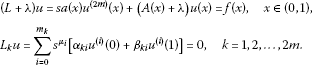(5)

Condition 1 Assume the following conditions are satisfied:

1. (1)

E is a UMD space, $p\in \left(1,\mathrm{\infty }\right)$;

2. (2)

$a\in \mathit{VMO}\cap {L}^{\mathrm{\infty }}\left(R\right)$, ${\eta }_{1}$ is a VMO modulus of a;

3. (3)

$|arg{\omega }_{j}-\pi |\le \frac{\pi }{2}-\phi$, $j=1,2,\dots ,m$, $|arg{\omega }_{j}|\le \frac{\pi }{2}-\phi$, $j=m+1,\dots ,2m$ and $\frac{\lambda }{{\omega }_{j}}\in S\left(\phi \right)$ for $0\le \phi <\pi$, $\eta \left(x\right)\ne 0$ a.e. $x\in \left[0,1\right]$;

4. (4)

$A\left(x\right)$ is a uniformly R-positive operator in E and

$A\left(\cdot \right){A}^{-1}\left({x}_{0}\right)\in {L}_{\mathrm{\infty }}\left(0,1;L\left(E\right)\right)\cap \mathit{VMO}\left(L\left(E\right)\right),\phantom{\rule{1em}{0ex}}{x}_{0}\in \left(0,1\right);$
5. (5)

$a\left(0\right)=a\left(1\right)$, $A\left(0\right)=A\left(1\right)$ and ${\eta }_{2}$ is a VMO modulus of $A\left(\cdot \right){A}^{-1}\left({x}_{0}\right)$.

First, we obtain an integral representation formula for solutions.

Lemma 1 Let Condition 1 hold and $f\in {L}^{p}\left(0,1;E\right)$. Then, for all solutions u of the problem (5) belonging to ${W}^{2m,p}\left(0,1;E\left(A\right),E\right)$, we have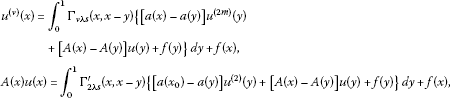(6)

where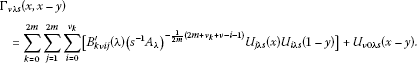Here ${B}_{kij}^{\mathrm{\prime }}\left(\lambda \right)$ are uniformly bounded operators and

${U}_{0\lambda s}\left(x-y\right)=\left\{\begin{array}{c}{a}^{-1}\left\{{s}^{1-\frac{1+\nu }{2m}}{A}_{\lambda }^{-\left(1-\frac{1-\nu }{2m}\right)}{\sum }_{i=1}^{m}{\left(-1\right)}^{2m+i}{\left({\omega }_{i}\right)}^{\nu }{P}_{i}^{-1}{U}_{i\lambda s}\left(x-y\right),x\ge y\right\},\hfill \\ -{a}^{-1}\left\{{s}^{1-\frac{1+\nu }{2m}}{A}_{\lambda }^{-\left(1-\frac{1-\nu }{2m}\right)}{\sum }_{i=m+1}^{2m}{\left(-1\right)}^{2m+\nu +i}{\left({\omega }_{i}\right)}^{\nu }{P}_{i}^{-1}{U}_{i\lambda s}\left(x-y\right),x\le y\right\},\hfill \end{array}$

and the expression ${\mathrm{\Gamma }}_{2\lambda }^{\mathrm{\prime }}\left(x,x-y\right)$ is a scalar multiple of ${\mathrm{\Gamma }}_{2\lambda }\left(x,x-y\right)$.

Proof Consider the problem (5) for $a\left(x\right)=a\left({x}_{0}\right)$ and $A\left(x\right)=A\left({x}_{0}\right)$, i.e.,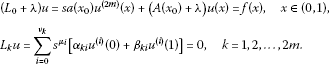(7)

Let $u\in {C}^{\left(2m\right)}\left(\left[0,1\right];E\left(A\right)\right)$ be a solution of the problem (7). Taking into account the equality ${L}_{0}u=\left({L}_{0}-L\right)u+Lu$ and Theorem A7, we get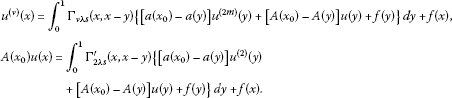Setting $x={x}_{0}$ in the above, we get (6) for $u\in {C}^{\left(2m\right)}\left(\left[0,1\right];E\left(A\right)\right)$. Then a density argument and Theorem A3 give the conclusion for

$u\in {W}^{2m,p}\left(0,1;E\left(A\right),E\right),\phantom{\rule{1em}{0ex}}{L}_{k}u=0.$

Consider the problem (5) on $\left(0,b\right)$, i.e.,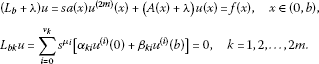(8)

□

Theorem 1 Suppose Condition 1 is satisfied. Then there exists a number $b\in \left(0,1\right)$ such that the following uniform coercive estimate holds:

$\sum _{i=0}^{2m}{s}^{\frac{i}{2m}}|\lambda {|}^{1-\frac{i}{2m}}{\parallel {u}^{\left(i\right)}\parallel }_{{L}^{p}\left(0,b;E\right)}+{\parallel Au\parallel }_{{L}^{p}\left(0,b;E\right)}\le C{\parallel \left({L}_{b}+\lambda \right)u\parallel }_{{L}^{p}\left(0,b;E\right)}$
(9)

for $u\in {W}^{2m,p}\left(0,b;E\left(A\right),E\right)$, $\lambda \in S\left(\phi \right)$ with large enough $|\lambda |$.

Proof By Lemma 1, for any solution $u\in {W}^{2m,p}\left(0,b;E\left(A\right),E\right)$ of the problem (8), we have

$\begin{array}{rcl}{u}^{\left(\nu \right)}\left(x\right)& =& {\int }_{0}^{b}{\mathrm{\Gamma }}_{\nu b\lambda s}\left(x,x-y\right)\left\{\left[a\left(x\right)-a\left(y\right)\right]{u}^{\left(2\right)}\left(y\right)\\ +\left[A\left(x\right)-A\left(y\right)\right]u\left(y\right)+f\left(y\right)\right\}\phantom{\rule{0.2em}{0ex}}dy+f\left(x\right),\end{array}$
(10)

where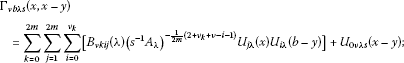(11)

here ${B}_{kij}\left(\lambda \right)$ are uniformly bounded operators, and

${U}_{0\nu \lambda s}\left(x-y\right)=\left\{\begin{array}{c}{a}^{-1}\left\{{s}^{1-\frac{1+\nu }{2m}}{A}_{\lambda }^{-\left(1-\frac{1-}{2m}\right)}{\sum }_{i=1}^{m}{\left(-1\right)}^{2m+i}{P}_{i}^{-1}{U}_{i\lambda s}\left(x-y\right),x\ge y\right\},\hfill \\ -{a}^{-1}\left\{{s}^{1-\frac{1}{2m}}{A}_{\lambda }^{-\left(1-\frac{1}{2m}\right)}{\sum }_{i=m+1}^{2m}{\left(-1\right)}^{2m+i}{P}_{i}^{-1}{U}_{i\lambda s}\left(x-y\right),x\le y\right\}.\hfill \end{array}$

Moreover, from (10) and (11), clearly, we get

$Au\left(x\right)={\int }_{0}^{b}{\mathrm{\Gamma }}_{b\lambda s}^{\mathrm{\prime }}\left(x,x-y\right)\left\{\left[a\left(x\right)-a\left(y\right)\right]{u}^{\left(2\right)}\left(y\right)+\left[A\left(x\right)-A\left(y\right)\right]u\left(y\right)+f\left(y\right)\right\}\phantom{\rule{0.2em}{0ex}}dy,$
(12)

where the expression ${\mathrm{\Gamma }}_{b\lambda }^{\mathrm{\prime }}\left(x,x-y\right)$ differs from ${\mathrm{\Gamma }}_{2b\lambda }\left(x,x-y\right)$ only by a constant.

Consider the operators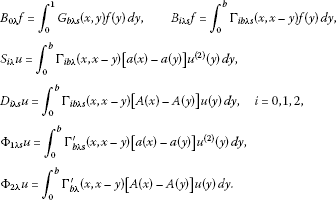Since the operators ${B}_{0\lambda }$ and ${B}_{1\lambda }$ are regular on ${L}^{p}\left(0,b;E\right)$, by using the positivity properties of A and the analyticity of semigroups ${U}_{k\lambda s}\left(x\right)$ in a similar way as in [, Theorem 3.1], we get

${\parallel {B}_{i\lambda }f\parallel }_{{L}^{p}\left(0,b;E\right)}\le M|\lambda {|}^{-\frac{2m-i}{2m}}{\parallel f\parallel }_{{L}^{p}\left(0,b;E\right)},\phantom{\rule{1em}{0ex}}i=0,1.$
(13)

Since the Hilbert operator is bounded in ${L}^{p}\left(R;E\right)$ for a UMD space E, we have

${\parallel {B}_{2\lambda }f\parallel }_{{L}^{p}\left(0,b;E\right)}\le M{\parallel f\parallel }_{{L}^{p}\left(0,b;E\right)}.$
(14)

Thus, by virtue of Theorems A4, A6 and in view of (10)-(12) for any $\epsilon >0$, there exists a positive number $b=b\left(\epsilon ,{\eta }_{1},{\eta }_{2}\right)$ such that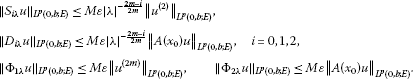(15)

Hence the estimates (13)-(15) imply (9). □

Theorem 2 Assume Condition 1 holds. Let $u\in {W}^{2m,p}\left(0,1;E\left(A\right),E\right)$ be a solution of (4). Then, for sufficiently large $|\lambda |$, $\lambda \in S\left(\phi \right)$, the following coercive uniform estimate holds:

$\sum _{i=0}^{2m}{s}^{\frac{i}{2m}}|\lambda {|}^{1-\frac{i}{2m}}{\parallel {u}^{\left(i\right)}\parallel }_{{L}^{p}\left(0,1;E\right)}+{\parallel Au\parallel }_{{L}^{p}\left(0,1;E\right)}\le C\left[{\parallel \left(L+\lambda \right)u\parallel }_{{L}^{p}\left(0,1;E\right)}+{\parallel u\parallel }_{{L}^{p}\left(0,1;E\right)}\right].$
(16)

Proof This fact is shown by covering and flattening argument, in a similar way as in Theorem A8. Particularly, by partition of unity, the problem is localized. Choosing diameters of supports for corresponding finite functions, by using Theorem 1, Theorems A4, A6, A7 and embedding Theorem A1 (see the same technique for DOEs with continuous coefficients [18, 19]), we obtain the assertion.

Let ${Q}_{s}$ denote the operator in ${L}^{p}\left(0,1;E\right)$ generated by the problem (4) for $\lambda =0$, i.e.,

$D\left({Q}_{s}\right)={W}^{2m,p}\left(0,1;E\left(A\right),E,{L}_{k}\right),\phantom{\rule{2em}{0ex}}{Q}_{s}u=sa\left(x\right){u}^{\left(2m\right)}+A\left(x\right)u.$

□

Theorem 3 Assume Condition 1 holds. Then, for all $f\in {L}^{p}\left(0,1;E\right)$, $\lambda \in S\left(\phi \right)$ and for large enough $|\lambda |$, the problem (5) has a unique solution $u\in {W}^{2m,p}\left(0,1;E\left(A\right),E\right)$. Moreover, the following coercive uniform estimate holds:

$\sum _{i=0}^{2m}{s}^{\frac{i}{2m}}|\lambda {|}^{1-\frac{i}{2m}}{\parallel {u}^{\left(i\right)}\parallel }_{{L}^{p}\left(0,1;E\right)}+{\parallel Au\parallel }_{{L}^{p}\left(0,1;E\right)}\le C{\parallel f\parallel }_{{L}^{p}\left(0,1;E\right)}.$
(17)

Proof First, let us show that the operator $Q+\lambda$ has a left inverse. Really, it is clear to see that

${\parallel u\parallel }_{{L}^{p}\left(0,1;E\right)}=\frac{1}{|\lambda |}\left[{\parallel \left({Q}_{s}+\lambda \right)u\parallel }_{{L}^{p}\left(0,1;E\right)}+{\parallel {Q}_{s}u\parallel }_{{L}^{p}\left(0,1;E\right)}\right].$

By Theorem A1 for $u\in {W}^{2m,p}\left(0,1;E\left(A\right),E\right)$, we have

${\parallel {Q}_{s}u\parallel }_{{L}^{p}\left(0,1;E\right)}\le C{\parallel u\parallel }_{{W}_{s}^{2m,p}\left(0,1;E\left(A\right),E\right)}.$

Then, by virtue of (16) and in view of the above relations, we infer for all $u\in {W}^{2m,p}\left(0,1;E\left(A\right),E\right)$ and sufficiently large $|\lambda |$ that there is a small ε and $C\left(\epsilon \right)$ such that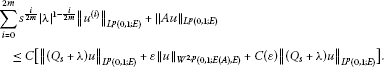(18)

In view of (18) for $u\in {W}^{2m,p}\left(0,1;E\left(A\right),E\right)$, we get

$\sum _{i=0}^{2m}{s}^{\frac{i}{2m}}|\lambda {|}^{1-\frac{i}{2m}}{\parallel {u}^{\left(i\right)}\parallel }_{{L}^{p}\left(0,1;E\right)}+{\parallel Au\parallel }_{{L}^{p}\left(0,1;E\right)}\le C{\parallel \left({Q}_{s}+\lambda \right)u\parallel }_{{L}^{p}\left(0,1;E\right)}.$
(19)

The estimate (19) implies that (4) has a unique solution and the operator ${Q}_{s}+\lambda$ has a bounded inverse in its rank space. We need to show that the rank space coincides with the all space ${L}^{p}\left(0,1;E\right)$. It suffices to prove that there is a solution $u\in {W}^{2m,p}\left(0,1;E\left(A\right),E\right)$ for all $f\in {L}^{p}\left(0,1;E\right)$. This fact can be derived in a standard way, approximating the equation with a similar one with smooth coefficients [18, 19]. More precisely, by virtue of [, Theorem 3.4], UMD spaces satisfy the multiplier condition. Moreover, by part (2) of Lemma A1, given $a\in \mathit{VMO}$ with VMO modules $\eta \left(r\right)$, we can find a sequence of mollifiers functions $\left\{{a}_{h}\right\}$ converging to a in BMO as $h\to 0$ with VMO modulus ${\eta }_{h}$ such that ${\eta }_{h}\left(r\right)\le \eta \left(r\right)$. In a similar way, it can be derived for the operator function $A\left(x\right){A}^{-1}\left({x}_{0}\right)\in \mathit{VMO}\left(L\left(E\right)\right)$. □

Result 1 Theorem 3 implies that the resolvent ${\left({Q}_{s}+\lambda \right)}^{-1}$ satisfies the following sharp uniform estimate:

$\sum _{i=0}^{2m}{s}^{\frac{i}{2m}}|\lambda {|}^{1-\frac{i}{2m}}{\parallel \frac{{d}^{i}}{d{x}^{i}}{\left({Q}_{s}+\lambda \right)}^{-1}\parallel }_{L\left({L}^{p}\left(0,1;E\right)\right)}+{\parallel A{\left({Q}_{s}+\lambda \right)}^{-1}\parallel }_{L\left({L}^{p}\left(0,1;E\right)\right)}\le C$
(20)

for $|arg\lambda |\le \phi$, $\phi \in \left(0,\pi \right)$ and $s>0$.

The estimate (20) particularly implies that the operator Q is uniformly positive in ${L}^{p}\left(0,1;E\right)$ and generates an analytic semigroup for $\phi \in \left(\frac{\pi }{2},\pi \right)$ (see, e.g., [, §1.14.5]).

Remark 1 Conditions $a\left(0\right)=a\left(1\right)$, $A\left(0\right)=A\left(1\right)$ arise due to nonlocality of the boundary conditions (4). If boundary conditions are local, then the conditions mentioned above are not required any more.

Consider the problem (1), where ${L}_{k}u$ is the same boundary condition as in (4). Let ${O}_{s}$ denote the differential operator generated by the problem (1). We will show the separability and Fredholmness of (1).

Theorem 4 Assume the following:

1. (1)

Condition 1 holds;

2. (2)

for any $\epsilon >0$, there is $C\left(\epsilon \right)>0$ such that for a.e. $x\in \left(0,1\right)$ and

${\parallel {A}_{k}\left(x\right)u\parallel }_{E}\le \epsilon {\parallel u\parallel }_{{\left(E\left(A\right),E\right)}_{\frac{k}{2m},\mathrm{\infty }}}+C\left(\epsilon \right)\parallel u\parallel ,\phantom{\rule{1em}{0ex}}u\in {\left(E\left(A\right),E\right)}_{\frac{1}{2},\mathrm{\infty }}.$

Then, for all $f\in {L}^{p}\left(0,1;E\right)$ and for large enough $|\lambda |$, $\lambda \in S\left(\phi \right)$, there is a unique solution $u\in {W}^{2m,p}\left(0,1;E\left(A\right),E\right)$ of the problem (1) and the following coercive uniform estimate holds:

$\sum _{i=0}^{2m}{s}^{\frac{i}{2m}}|\lambda {|}^{1-\frac{i}{2m}}{\parallel {u}^{\left(i\right)}\parallel }_{{L}^{p}\left(0,1;E\right)}+{\parallel Au\parallel }_{{L}^{p}\left(0,1;E\right)}\le C{\parallel f\parallel }_{{L}^{p}\left(0,1;E\right)}.$
(21)

Proof It is sufficient to show that the operator ${O}_{s}+\lambda$ has a bounded inverse ${\left({O}_{s}+\lambda \right)}^{-1}$ from ${L}^{p}\left(0,1;E\right)$ to ${W}^{2m,p}\left(0,1;E\left(A\right),E\right)$. Put ${O}_{s}u={Q}_{s}u+{Q}_{1}u$, where

${Q}_{1}u=\sum _{k=0}^{2m-1}{s}^{\frac{k}{2m}}{A}_{k}\left(x\right){u}^{\left(k\right)}\left(x\right),\phantom{\rule{1em}{0ex}}u\in {W}^{2m,p}\left(0,1;E\left(A\right),E,{L}_{k}\right).$

By the second assumption and Theorem A1, there is a small ε and $C\left(\epsilon \right)$ such that

$\begin{array}{rcl}{\parallel {s}^{\frac{k}{2m}}{A}_{k}{u}^{\left(k\right)}\parallel }_{{L}^{p}\left(0,1;E\right)}& \le & C{\parallel {s}^{\frac{k}{2m}}{A}^{1-\frac{k}{2m}}u\parallel }_{{L}^{p}\left(0,1;E\right)}\\ \le & \epsilon {\parallel u\parallel }_{{W}_{s}^{2m,p}\left(0,1;E\left(A\right),E\right)}+C\left(\epsilon \right){\parallel u\parallel }_{{L}^{p}\left(0,1;E\right)}.\end{array}$
(22)

By Theorem 3, the operator ${Q}_{s}+\lambda$ has a bounded inverse ${\left({Q}_{s}+\lambda \right)}^{-1}$ from ${L}^{p}\left(0,1;E\right)$ to ${W}^{2m,p}\left(0,1;E\left(A\right),E\right)$ for sufficiently large $|\lambda |$. So, (22) implies the following uniform estimate:

${\parallel {Q}_{1}{\left({Q}_{s}+\lambda \right)}^{-1}\parallel }_{L\left({L}^{p}\left(0,1;E\right)\right)}<1.$

It is clear to see that

$\left({O}_{s}+\lambda \right)=\left[I+{Q}_{1}{\left({Q}_{s}+\lambda \right)}^{-1}\right]\left({Q}_{s}+\lambda \right),\phantom{\rule{2em}{0ex}}{\left({O}_{s}+\lambda \right)}^{-1}={\left(Q+\lambda \right)}^{-1}{\left[I+{Q}_{1}{\left({Q}_{s}+\lambda \right)}^{-1}\right]}^{-1}.$

Then, by the above relation and by virtue of Theorem 3, we get the assertion. □

Theorem 4 implies the following result.

Result 2 Suppose all conditions of Theorem 4 are satisfied. Then the resolvent ${\left({O}_{s}+\lambda \right)}^{-1}$ of the operator ${O}_{s}$ satisfies the following sharp uniform estimate:

$\sum _{i=0}^{2m}{s}^{\frac{i}{2m}}|\lambda {|}^{1-\frac{i}{2m}}{\parallel \frac{{d}^{i}}{d{x}^{i}}{\left({O}_{s}+\lambda \right)}^{-1}\parallel }_{L\left({L}^{p}\left(0,1;E\right)\right)}+{\parallel A{\left({O}_{s}+\lambda \right)}^{-1}\parallel }_{L\left({L}^{p}\left(0,1;E\right)\right)}\le C$

for $|arg\lambda |\le \phi$, $\phi \in \left[0\pi \right)$ and $s>0$.

Consider the problem (1) for $\lambda =0$, i.e.,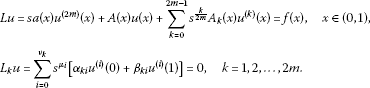(23)

Theorem 5 Assume all conditions of Theorem 4 hold and ${A}^{-1}\in {\sigma }_{\mathrm{\infty }}\left(E\right)$. Then the problem (23) is Fredholm from ${W}^{2m,p}\left(0,1;E\left(A\right),E\right)$ into ${L}^{p}\left(0,1;E\right)$.

Proof Theorem 4 implies that the operator ${O}_{s}+\lambda$ has a bounded inverse ${\left({O}_{s}+\lambda \right)}^{-1}$ from ${L}^{p}\left(0,1;E\right)$ to ${W}^{2m,p}\left(0,1;E\left(A\right),E\right)$ for large enough $|\lambda |$; that is, the operator ${O}_{s}+\lambda$ is Fredholm from ${W}^{2m,p}\left(0,1;E\left(A\right),E\right)$ into ${L}^{p}\left(0,1;E\right)$. Then, by virtue of Theorem A2 and by perturbation theory of linear operators, we obtain the assertion. □

## 4 Nonlinear DOEs with VMO coefficients

Let at first consider the linear BVP in a moving domain $\left(0,b\left(s\right)\right)$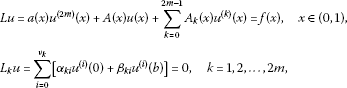(24)

where a is a complex-valued function and $A=A\left(x\right)$, ${A}_{k}={A}_{k}\left(x\right)$ are linear operators in a Banach space E, where $b\left(s\right)$ is a positive continuous function independent of u.

Theorem 4 implies the following.

Result 3 Let all conditions of Theorem 4 be satisfied. Then the problem (24) has a unique solution $u\in {W}^{2m,p}\left(0,b;E\left(A\right),E\right)$ for $f\in {L}^{p}\left(0,b\left(s\right);E\right)$, $p\in \left(1,\mathrm{\infty }\right)$, $\lambda \in {S}_{\phi }$ with large enough $|\lambda |$, and the following coercive uniform estimate holds:

$\sum _{i=0}^{2m}|\lambda {|}^{1-\frac{i}{2m}}{\parallel {u}^{\left(i\right)}\parallel }_{{L}^{p}\left(0,b;E\right)}+{\parallel Au\parallel }_{{L}^{p}\left(0,b;E\right)}\le {\parallel f\parallel }_{{L}^{p}\left(0,b;E\right)}.$

Proof Really, under the substitution $\tau =xb\left(s\right)$, the moving boundary problem (24) maps to the following BVP with parameter in the fixed domain $\left(0,1\right)$: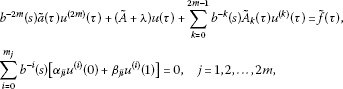where

$\tau \in \left(0,1\right),\phantom{\rule{1em}{0ex}}\stackrel{˜}{A}=A\left(\tau {b}^{-1}\left(s\right)\right),\phantom{\rule{2em}{0ex}}{\stackrel{˜}{A}}_{k}={A}_{k}\left(\tau {b}^{-1}\left(s\right)\right),\stackrel{˜}{f}\left(\tau \right)=f\left(\tau {b}^{-1}\left(s\right)\right).$

Then, by virtue of Theorem 4, we obtain the assertion. □

Consider the following nonlinear problem: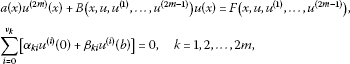(25)

where ${\nu }_{k}\in \left\{0,1,\dots ,2m-1\right\}$, ${\alpha }_{ki}$, ${\beta }_{ki}$ are complex numbers, $x\in \left(0,b\right)$, where b is a positive number in $\left(0,{b}_{0}\right]$.

In this section, we will prove the existence and uniqueness of a maximal regular solution of the nonlinear problem (25). Assume A is a φ-positive operator in a Banach space E. Let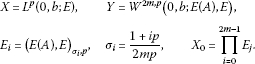Remark 2 By using [, §1.8.], we obtain that the embedding ${D}^{j}Y\in {E}_{j}$ is continuous and there exists a constant ${C}_{1}$ such that for $w\in Y$, $W=\left\{{w}_{0,}{w}_{1},\dots ,{w}_{2m-1}\right\}$, ${w}_{j}={D}^{j}w\left(\cdot \right)$,

${\parallel w\parallel }_{{X}_{0},\mathrm{\infty }}=\prod _{i=0}^{2m-1}{\parallel {D}^{i}w\parallel }_{C\left(\left[0,b\right],{E}_{j}\right)}=\underset{x\in \left[0,b\right]}{sup}\prod _{i=0}^{1}{\parallel {D}^{i}w\left(x\right)\parallel }_{{E}_{j}}\le {C}_{1}{\parallel w\parallel }_{Y}.$

Condition 2 Assume the following are satisfied:

1. (1)

E is a UMD space, $p\in \left(1,\mathrm{\infty }\right)$;

2. (2)

$a\in \mathit{VMO}\cap {L}^{\mathrm{\infty }}\left(R\right)$, $a\left(0\right)=a\left(b\right)$;

3. (3)

$|arg{\omega }_{j}-\pi |\le \frac{\pi }{2}-\phi$, $j=1,2,\dots ,m$, $|arg{\omega }_{j}|\le \frac{\pi }{2}-\phi$, $j=m+1,\dots ,2m$ and $\frac{\lambda }{{\omega }_{j}}\in S\left(\phi \right)$ for $\lambda \in S\left(\phi \right)$, $0\le \phi <\pi$, $\eta \left(x\right)\ne 0$ a.e. $x\in \left[0,1\right]$;

4. (3)

$F\left(x,{\upsilon }_{0},{\upsilon }_{1},\dots ,{\upsilon }_{2m-1}\right):\left[0,b\right]×{X}_{0}\to E$ is a measurable function for each ${\upsilon }_{i}\in {E}_{i}$, $i=0,1,\dots ,2m-1$; $F\left(x,\cdot ,\cdot \right)$ is continuous with respect to $x\in \left[0,b\right]$ and $f\left(x\right)=F\left(x,0\right)\in X$. Moreover, for each $R>0$, there exists ${\mu }_{R}$ such that

${\parallel F\left(x,U\right)-F\left(x,\overline{U}\right)\parallel }_{E}\le {\mu }_{R}{\parallel U-\overline{U}\parallel }_{{X}_{0}},$

where $U=\left\{{u}_{0},{u}_{1},\dots ,{u}_{2m-1}\right\}$ and $\overline{U}=\left\{{\overline{u}}_{0},{\overline{u}}_{1},\dots ,{\overline{u}}_{2m-1}\right\}$ for a.a. $x\in \left[0,b\right]$, ${u}_{i},{\overline{u}}_{i}\in {E}_{i}$ and

${\parallel U\parallel }_{{X}_{0}}\le R,{\parallel \overline{U}\parallel }_{{X}_{0}}\le R.$
1. (4)

for $U\in {X}_{0}$, the operator $B\left(x,U\right)$ is R-positive in E uniformly with respect to $x\in \left[0,b\right]$; $B\left(x,U\right){B}^{-1}\left({x}^{0},U\right)\in {L}_{\mathrm{\infty }}\left(0,1;L\left(E\right)\right)\cap \mathit{VMO}\left(L\left(E\right)\right)$, ${x}_{0}\in \left(0,1\right)$, where domain definition $D\left(B\left(x,U\right)\right)$ does not depend on x and U; $B\left(x,W\right):\left(0,b\right)×{X}_{0}\to B\left(E\left(A\right),E\right)$ is continuous, where $A=A\left(x\right)=B\left(x,W\right)$ for fixed $W=\left\{{w}_{0},{w}_{1},\dots ,{w}_{2m-1}\right\}\in {X}_{0}$;

2. (5)

for each $R>0$, there is a positive constant $L\left(R\right)$ such that ${\parallel \left[B\left(x,U\right)-B\left(x,\overline{U}\right)\right]\upsilon \parallel }_{E}\le L\left(R\right){\parallel U-\overline{U}\parallel }_{{X}_{0}}{\parallel A\upsilon \parallel }_{E}$ for $x\in \left(0,b\right)$, $U,\overline{U}\in {X}_{0}$, ${\parallel U\parallel }_{{X}_{0}},{\parallel \overline{U}\parallel }_{{X}_{0}}\le R$ and $\upsilon \in D\left(A\right)$ and $A\left(0\right)=A\left(b\right)$.

Theorem 6 Let Condition 2 hold. Then there is $b\in \left(0,{b}_{0}\right]$ such that the problem (26) has a unique solution belonging to space ${W}^{2m,p}\left(0,b;E\left(A\right),E\right)$.

Proof

Consider the linear problem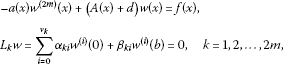(26)

where

$f\left(x\right)=F\left(x,0\right),\phantom{\rule{1em}{0ex}}{x}_{0}\in \left(0,b\right).$

By virtue of Result 3, the problem (26) has a unique solution for all $f\in X$ and for sufficiently large $d>0$ that satisfies the following:

${\parallel w\parallel }_{Y}\le {C}_{0}{\parallel f\parallel }_{X},$

where the constant C does not depend on $f\in X$ and $b\in \left(0,{b}_{0}\right]$. We want to solve the problem (25) locally by means of maximal regularity of the linear problem (26) via the contraction mapping theorem. For this purpose, let w be a solution of the linear BVP (27). Consider a ball

${B}_{r}=\left\{\upsilon \in Y,{L}_{k}\upsilon =0,k=1,2,\dots ,2m,{\parallel \upsilon -w\parallel }_{Y}\le r\right\}.$

For $\upsilon \in {B}_{r}$, consider the linear problem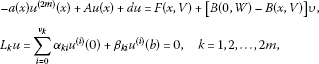(27)

where

$V=\left\{\upsilon ,{\upsilon }^{\left(1\right)},\dots ,{\upsilon }^{\left(2m-1\right)}\right\},\phantom{\rule{2em}{0ex}}W=\left\{w,{w}^{\left(1\right)},\dots ,{w}^{\left(2m-1\right)}\right\}.$

Define a map Q on ${B}_{r}$ by $Q\upsilon =u$, where u is a solution of the problem (27). We want to show that $Q\left({B}_{r}\right)\subset {B}_{r}$ and that Q is a contraction operator provided b is sufficiently small and r is chosen properly. For this aim, by using maximal regularity properties of the problem (28), we have

${\parallel Q\upsilon -w\parallel }_{Y}={\parallel u-w\parallel }_{Y}\le {C}_{0}\left\{{\parallel F\left(x,V\right)-F\left(x,0\right)\parallel }_{X}+{\parallel \left[B\left(0,W\right)-B\left(x,V\right)\right]\upsilon \parallel }_{X}\right\}.$

By assumption (5), we have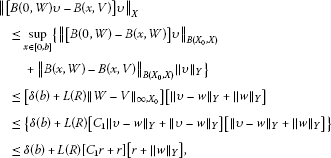where

$\delta \left(b\right)=\underset{x\in \left[0,b\right]}{sup}{\parallel \left[B\left(0,W\right)-B\left(x,W\right)\right]\parallel }_{B\left({X}_{0},X\right)}.$

Bear in mind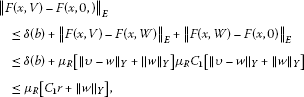where $R={C}_{1}r+{\parallel w\parallel }_{Y}$ is a fixed number. In view of the above estimates, by a suitable choice of ${\mu }_{R}$, ${L}_{R}$ and for sufficiently small $b\in \left[0;{b}_{0}\right)$, we have

${\parallel Q\upsilon -w\parallel }_{Y}\le r,$

i.e.,

$Q\left({B}_{r}\right)\subset {B}_{r}.$

Moreover, in a similar way, we obtain

$\begin{array}{rcl}{\parallel Q\upsilon -Q\overline{\upsilon }\parallel }_{Y}& \le & {C}_{0}\left\{{\mu }_{R}{C}_{1}+{M}_{a}+L\left(R\right)\left[{\parallel \upsilon -w\parallel }_{Y}+{C}_{1}r\right]\\ +L\left(R\right){C}_{1}\left[r+{\parallel w\parallel }_{Y}\right]{\parallel \upsilon -\overline{\upsilon }\parallel }_{Y}\right\}+\delta \left(b\right).\end{array}$

By a suitable choice of ${\mu }_{R}$, ${L}_{R}$ and for sufficiently small $b\in \left(0,{b}_{0}\right)$, we obtain ${\parallel Q\upsilon -Q\overline{\upsilon }\parallel }_{Y}<\eta {\parallel \upsilon -\overline{\upsilon }\parallel }_{Y}$, $\eta <1$, i.e., Q is a contraction operator. Eventually, the contraction mapping principle implies a unique fixed point of Q in ${B}_{r}$ which is the unique strong solution $u\in Y$. □

## 5 Boundary value problems for anisotropic elliptic equations with VMO coefficients

The Fredholm property of BVPs for elliptic equations with parameters in smooth domains were studied, e.g., in [14, 24, 28]; also, for non-smooth domains, these questions were investigated, e.g., in .

Let $\mathrm{\Omega }\subset {R}^{n}$ be an open connected set with compact ${C}^{2l}$-boundary Ω. Let us consider the nonlocal boundary value problems on a cylindrical domain $G=\left(0,1\right)×\mathrm{\Omega }$ for the following anisotropic elliptic equation with VMO top-order coefficients: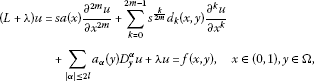(28)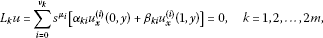(29)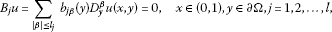(30)

where s is a positive parameter, a, ${d}_{i}$ are complex-valued functions, ${\alpha }_{ki}$ and ${\beta }_{ki}$ are complex numbers,

${D}_{j}=-i\frac{\partial }{\partial {y}_{j}},\phantom{\rule{2em}{0ex}}{\nu }_{k}\in \left\{0,1,\dots ,2m-1\right\},\phantom{\rule{2em}{0ex}}y=\left({y}_{1},\dots ,{y}_{n}\right),\phantom{\rule{2em}{0ex}}{\mu }_{i}=\frac{i}{2m}+\frac{1}{2mp}.$

For $G=\left(0,1\right)×\mathrm{\Omega }$, $\mathbf{p}=\left({p}_{1},p\right)$, ${L}^{\mathbf{p}}\left(G\right)$ will denote the space of all p-summable scalar-valued functions with a mixed norm (see, e.g., [, §1]), i.e., the space of all measurable functions f defined on G, for which

${\parallel f\parallel }_{{L}^{\mathbf{p}}\left(G\right)}={\left({\int }_{0}^{1}{\left({\int }_{\mathrm{\Omega }}|f\left(x,y\right){|}^{{p}_{1}}\phantom{\rule{0.2em}{0ex}}dy\right)}^{\frac{p}{{p}_{1}}}\phantom{\rule{0.2em}{0ex}}dx\right)}^{\frac{1}{p}}<\mathrm{\infty }.$

Analogously, ${W}^{2m,2l,\mathbf{p}}\left(G\right)$ denotes the anisotropic Sobolev space with the corresponding mixed norm [, §10].

Theorem 7 Let the following conditions be satisfied:

1. (1)

$a,{d}_{0}\in \mathit{VMO}\cap {L}^{\mathrm{\infty }}\left(R\right)$, $a\left(0\right)=a\left(1\right)$;

2. (2)

$|arg{\omega }_{j}-\pi |\le \frac{\pi }{2}-\phi$, $j=1,2,\dots ,m$, $|arg{\omega }_{j}|\le \frac{\pi }{2}-\phi$, $j=m+1,\dots ,2m$ and $\frac{\lambda }{{\omega }_{j}}\in S\left(\phi \right)$ for $\lambda \in S\left(\phi \right)$, $0\le \phi <\pi$, $\eta \left(x\right)\ne 0$, a.e. $x\in \left[0,1\right]$;

3. (3)

${d}_{k}\in {L}^{\mathrm{\infty }}$, ${d}_{k}\left(\cdot ,y\right){d}_{0}^{1-\frac{k}{2m}-{\sigma }_{k}}\left(\cdot \right)\in {L}^{\mathrm{\infty }}\left(0,1\right)$ for a.e. $y\in \mathrm{\Omega }$ and $0<{\sigma }_{k}<1-\frac{k}{2m}$;

4. (4)

${a}_{\alpha }\in \mathit{VMO}\cap {L}^{\mathrm{\infty }}\left({R}^{n}\right)$ for each $|\alpha |=2l$ and ${a}_{\alpha }\in \left[{L}^{\mathrm{\infty }}+{L}^{{\gamma }_{k}}\right]\left(\mathrm{\Omega }\right)$ for each $|\alpha |=k<2l$ with ${r}_{k}\ge q$ and $2l-k>\frac{l}{{r}_{k}}$;

5. (5)

${b}_{j\beta }\in {C}^{2l-{l}_{j}}\left(\partial \mathrm{\Omega }\right)$ for each j, β and ${m}_{j}<2l$, ${\sum }_{j=1}^{l}{b}_{j\beta }\left({y}^{\mathrm{\prime }}\right){\sigma }_{j}\ne 0$ for $|\beta |={l}_{j}$, ${y}^{\mathrm{\prime }}\in \partial G$, where $\sigma =\left({\sigma }_{1},{\sigma }_{2},\dots ,{\sigma }_{n}\right)\in {R}^{n}$ is a normal to ∂G;

6. (6)

for $y\in \overline{\mathrm{\Omega }}$, $\xi \in {R}^{n}$, $\nu \in S\left(\phi \right)$, $\phi \in \left(0,\pi \right)$, $|\xi |+|\nu |\ne 0$ let $\nu +{\sum }_{|\alpha |=2l}{a}_{\alpha }\left(y\right){\xi }^{\alpha }\ne 0$;

7. (7)

for each ${y}_{0}\in \partial \mathrm{\Omega }$, the local BVP in local coordinates corresponding to ${y}_{0}$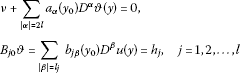has a unique solution $\vartheta \in {C}_{0}\left({R}_{+}\right)$ for all $h=\left({h}_{1},{h}_{2},\dots ,{h}_{n}\right)\in {R}^{n}$, and for ${\xi }^{\mathrm{\prime }}\in {R}^{n-1}$ with $|{\xi }^{\mathrm{\prime }}|+|\nu |\ne 0$.

Then

1. (a)

for all $f\in {L}^{\mathbf{p}}\left(G\right)$, $\lambda \in S\left(\phi \right)$ and sufficiently large $|\lambda |$, the problem (28)-(30) has a unique solution u belonging to ${W}^{2m,2l,\mathbf{p}}\left(G\right)$ and the following coercive uniform estimate holds:

$\sum _{i=0}^{2m}{s}^{\frac{i}{2m}}|\lambda {|}^{1-\frac{i}{2m}}{\parallel \frac{{\partial }^{i}u}{{\partial }^{i}x}\parallel }_{{L}^{\mathbf{p}}\left(G\right)}+\sum _{|\beta |=2l}{\parallel {D}_{y}^{\beta }u\parallel }_{{L}^{\mathbf{p}}\left(G\right)}\le C{\parallel f\parallel }_{{L}^{\mathbf{p}}\left(G\right)};$
2. (b)

for $\lambda =0$ the problem (28)-(30) is Fredholm in ${L}^{\mathbf{p}}\left(G\right)$.

Proof Let $E={L}^{{p}_{1}}\left(\mathrm{\Omega }\right)$. Then by virtue of , part (1) of Condition 1 is satisfied. Consider the operator A acting in ${L}^{{p}_{1}}\left(\mathrm{\Omega }\right)$ defined by

$D\left(A\right)={W}^{2l,{p}_{1}}\left(\mathrm{\Omega };{B}_{j}u=0\right),\phantom{\rule{2em}{0ex}}Au=\sum _{|\alpha |\le 2l}{a}_{\alpha }\left(y\right){D}^{\alpha }u\left(y\right).$

For $x\in \mathrm{\Omega }$ also consider operators in ${L}^{{p}_{1}}\left(\mathrm{\Omega }\right)$

$D\left({A}_{k}\right)={W}^{2l,{p}_{1}}\left(\mathrm{\Omega };{B}_{j}u=0\right),\phantom{\rule{2em}{0ex}}{A}_{k}\left(x\right)u={d}_{k}\left(x,y\right)u\left(y\right).$

The problem (28)-(30) can be rewritten in the form (1), where $u\left(x\right)=u\left(x,\cdot \right)$, $f\left(x\right)=f\left(x,\cdot \right)$ are functions with values in $E={L}^{{p}_{1}}\left(\mathrm{\Omega }\right)$. By virtue of [, Theorem 8.2], the problem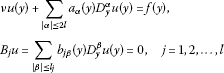has a unique solution for $f\in {L}^{{p}_{1}}\left(\mathrm{\Omega }\right)$ and $arg\nu \in S\left(\phi \right)$, $|\nu |\to \mathrm{\infty }$, and the operator A is R-positive in ${L}^{{p}_{1}}\left(\mathrm{\Omega }\right)$, i.e., Condition 1 holds. Moreover, it is known that the embedding ${W}^{2l,{p}_{1}}\left(\mathrm{\Omega }\right)\subset {L}^{{p}_{1}}\left(\mathrm{\Omega }\right)$ is compact (see, e.g., [, Theorem 3.2.5]). Then, by using interpolation properties of Sobolev spaces (see, e.g., [, §4]), it is clear to see that condition (2) of Theorem 4 is fulfilled too. Then from Theorems 4, 5 the assertions are obtained. □

## 6 Systems of differential equations with VMO coefficients

Consider the nonlocal BVPs for infinity systems of parameter-differential equations with principal VMO coefficients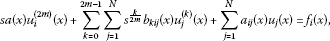(31)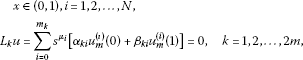(32)

where s is a positive parameter, a, ${b}_{mj}$, ${d}_{mj}$ are complex-valued functions, N is finite or infinite natural number, ${\alpha }_{ki}$ and ${\beta }_{ki}$ are complex numbers, ${\mu }_{i}=\frac{i}{2m}+\frac{1}{2mp}$.

Let ${a}_{ij}\left(x\right)$ be a real function and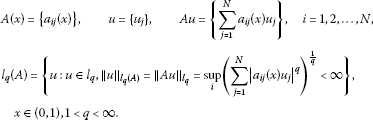From Theorem 4, we obtain the following.

Theorem 8 Suppose the following conditions are satisfied:

1. (1)

$a\in \mathit{VMO}\cap {L}^{\mathrm{\infty }}\left(R\right)$, $a\left(0\right)=a\left(1\right)$;

2. (2)

$|arg{\omega }_{j}-\pi |\le \frac{\pi }{2}-\phi$, $j=1,2,\dots ,m$, $|arg{\omega }_{j}|\le \frac{\pi }{2}-\phi$, $j=m+1,\dots ,2m$ and $\frac{\lambda }{{\omega }_{j}}\in S\left(\phi \right)$ for $0\le \phi <\pi$, $\eta \left(x\right)\ne 0$ a.e. $x\in \left(0,1\right)$;

3. (3)

${a}_{ij}\ne 0$ and ${a}_{ij}={a}_{ji}$, ${a}_{ij}\in \mathit{VMO}\cap {L}^{\mathrm{\infty }}\left(R\right)$, $p\in \left(1,\mathrm{\infty }\right)$.

Then, for all $f\left(x\right)={\left\{{f}_{m}\left(x\right)\right\}}_{1}^{N}\in {L}^{p}\left(0,1;{l}_{q}\right)$, $\lambda \in S\left(\phi \right)$ and for sufficiently large $|\lambda |$, problem (32)-(33) has a unique solution $u={\left\{{u}_{m}\left(x\right)\right\}}_{1}^{\mathrm{\infty }}$ belonging to ${W}^{2m,p}\left(\left(0,1\right),{l}_{q}\left(D\right),{l}_{q}\right)$ and the following coercive estimate holds:

$\sum _{i=0}^{2m}{s}^{\frac{i}{2m}}|\lambda {|}^{1-\frac{i}{2m}}{\parallel {u}^{\left(i\right)}\parallel }_{{L}^{p}\left(0,1;{l}_{q}\right)}+{\parallel Au\parallel }_{{L}^{p}\left(0,1;{l}_{q}\right)}\le C{\parallel f\parallel }_{{L}^{p}\left(0,1;{l}_{q}\right)}.$
(33)

Proof Really, let $E={l}_{q}$, A and ${A}_{k}\left(x\right)$ be matrices such that

$A=\left[{d}_{ij}\left(x\right)\right],\phantom{\rule{2em}{0ex}}{A}_{k}\left(x\right)=\left[{b}_{kij}\left(x\right){\delta }_{ij}\right],\phantom{\rule{1em}{0ex}}i,j=1,2,\dots ,N.$

It is clear to see that the operator A is R-positive in ${l}_{q}$. Therefore, by Theorem 4, the problem (31)-(32) has a unique solution $u\in {W}^{2m,p}\left(\left(0,1\right);{l}_{q}\left(A\right),{l}_{q}\right)$ for all $f\in {L}^{p}\left(\left(0,1\right);{l}_{q}\right)$, $\lambda \in S\left(\phi \right)$ and the estimate (33) holds. □

Remark 3 There are many positive operators in different concrete Banach spaces. Therefore, putting concrete Banach spaces and concrete positive operators (i.e., pseudo-differential operators or finite or infinite matrices for instance) instead of E and A, respectively, by virtue of Theorems 4 and 5, we can obtain a different class of maximal regular BVPs for partial differential or pseudo-differential equations or its finite and infinite systems with VMO coefficients.

## References

1. Sarason D: On functions of vanishing mean oscillation. Trans. Am. Math. Soc. 1975, 207: 391–405.

2. Chiarenza F, Frasca M, Longo P: ${W}^{2,p}$-solvability of the Dirichlet problem for nondivergence elliptic equations with VMO coefficients. Trans. Am. Math. Soc. 1993, 336(2):841–853.

3. Chiarenza F: ${L}^{p}$ regularity for systems of PDEs with coefficients in VMO. Nonlinear Analysis, Function Spaces and Applications, Vol. 5 (Prague, 1994) 1994.

4. Chiarenza F, Frasca M, Longo P: Interior ${W}^{2,p}$ estimates for non divergence elliptic equations with discontinuous coefficients. Ric. Mat. 1991, 40: 149–168.

5. Miranda C: Partial Differential Equations of Elliptic Type. Springer, Berlin; 1970.

6. Maugeri A, Palagachev DK, Softova L: Elliptic and Parabolic Equations with Discontinuous Coefficients. Wiley, Berlin; 2000.

7. Krylov NV: Parabolic and elliptic equations with VMO coefficients. Commun. Partial Differ. Equ. 2007, 32(3):453–475. 10.1080/03605300600781626

8. Palagachev DK, Recke L, Softova L: Applications of the differential calculus to nonlinear elliptic operators with discontinuous coefficients. Math. Ann. 2006, 336(3):617–637. 10.1007/s00208-006-0014-x

9. Ragusa MA: Necessary and sufficient condition for VMO function. Appl. Math. Comput. 2012, 218(24):11952–11958. 10.1016/j.amc.2012.06.005

10. Ragusa MA: Embeddings for Morrey-Lorentz spaces. J. Optim. Theory Appl. 2012, 154(2):491–499. 10.1007/s10957-012-0012-y

11. Heck H, Hieber M: Maximal ${L}^{p}$-regularity for elliptic operators with VMO-coefficients. J. Evol. Equ. 2003, 3: 62–88.

12. Amann H 1. In Linear and Quasi-Linear Equations. Birkhäuser, Basel; 1995.

13. Ashyralyev A: On well-posedness of the nonlocal boundary value problem for elliptic equations. Numer. Funct. Anal. Optim. 2003, 24(1–2):1–15. 10.1081/NFA-120020240

14. Denk R, Hieber M, Prüss J: R -boundedness, Fourier multipliers and problems of elliptic and parabolic type. Mem. Am. Math. Soc. 2003., 166: Article ID 788

15. Favini A, Shakhmurov V, Yakubov Y: Regular boundary value problems for complete second order elliptic differential-operator equations in UMD Banach spaces. Semigroup Forum 2009., 79: Article ID 1

16. Gorbachuk VI, Gorbachuk ML: Boundary Value Problems for Differential-Operator Equations. Naukova Dumka, Kiev; 1984.

17. Lions JL, Peetre J: Sur une classe d’espaces d’interpolation. Publ. Math. 1964, 19: 5–68.

18. Shakhmurov VB: Coercive boundary value problems for regular degenerate differential-operator equations. J. Math. Anal. Appl. 2004, 292(2):605–620. 10.1016/j.jmaa.2003.12.032

19. Shakhmurov VB: Separable anisotropic differential operators and applications. J. Math. Anal. Appl. 2007, 327(2):1182–1201. 10.1016/j.jmaa.2006.05.007

20. Shakhmurov VB, Shahmurova A: Nonlinear abstract boundary value problems atmospheric dispersion of pollutants. Nonlinear Anal., Real World Appl. 2010, 11: 932–951. 10.1016/j.nonrwa.2009.01.037

21. Shakhmurov VB: Embedding theorems and maximal regular differential operator equations in Banach-valued function spaces. J. Inequal. Appl. 2005, 4: 605–620.

22. Shakhmurov VB: Linear and nonlinear abstract equations with parameters. Nonlinear Anal., Theory Methods Appl. 2010, 73: 2383–2397. 10.1016/j.na.2010.06.004

23. Weis L: Operator-valued Fourier multiplier theorems and maximal ${L}_{p}$ regularity. Math. Ann. 2001, 319: 735–758. 10.1007/PL00004457

24. Yakubov S, Yakubov Y: Differential-Operator Equations. Ordinary and Partial Differential Equations. Chapman & Hall/CRC, Boca Raton; 2000.

25. John F, Nirenberg L: On functions of bounded mean oscillation. Commun. Pure Appl. Math. 1961, 14: 415–476. 10.1002/cpa.3160140317

26. Segovia C, Torrea JL: Vector-valued commutators and applications. Indiana Univ. Math. J. 1989, 38(4):959–971. 10.1512/iumj.1989.38.38044

27. Burkholder DL: A geometric condition that implies the existence of certain singular integrals of Banach-space-valued functions. In Conference on Harmonic Analysis in Honor of Antoni Zygmund, Vol. I, II. Wadsworth, Belmont; 1983:270–286. Chicago, Ill., 1981

28. Triebel H: Interpolation Theory, Function Spaces, Differential Operators. North-Holland, Amsterdam; 1978.

29. Blasco O: Operator valued BMO commutators. Publ. Mat. 2009, 53(1):231–244.

30. Hytönen T, Weis L: A T1 theorem for integral transformations with operator-valued kernel. J. Reine Angew. Math. 2006, 599: 155–200.

31. Grisvard P: Elliptic Problems in Nonsmooth Domains. Pitman, London; 1985.

32. Besov OV, Ilin VP, Nikolskii SM: Integral Representations of Functions and Embedding Theorems. Nauka, Moscow; 1975.

## Acknowledgements

Dedicated to Professor Hari M Srivastava.

## Author information

Authors

### Corresponding author

Correspondence to Veli Shakhmurov.

### Competing interests

The author declares that they have no competing interests.

## Rights and permissions

Reprints and Permissions

Shakhmurov, V. Optimal regular differential operators with variable coefficients and applications. Fixed Point Theory Appl 2013, 42 (2013). https://doi.org/10.1186/1687-1812-2013-42

• Accepted:

• Published:

• DOI: https://doi.org/10.1186/1687-1812-2013-42

### Keywords

• differential equations with VMO coefficients
• boundary value problems
• differential-operator equations
• maximal ${L}^{p}$ regularity
• abstract function spaces
• nonlinear elliptic equations